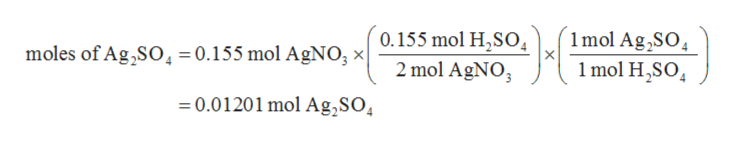# If 0.155 moles of AgNO₃ react with 0.155 moles of H₂SO₄ according to this UNBALANCED equation below, how many grams of Ag₂SO₄ could be formed? AgNO₃(aq) + H₂SO₄ (aq) → Ag₂SO₄ (s) + HNO₃ (aq)

Question
849 views

If 0.155 moles of AgNO₃ react with 0.155 moles of H₂SO₄ according to this UNBALANCED equation below, how many grams of Ag₂SO₄ could be formed? AgNO₃(aq) + H₂SO₄ (aq) → Ag₂SO₄ (s) + HNO₃ (aq)

check_circle

Step 1

The values are given as follows:

Step 2

The balanced chemical equation can be written as follows:

Step 3

Since, 2 moles of AgNO3 react with 1 mole of H2SO4 in order to produce 1 mole of Ag2SO4.  Therefore, the numbe...help_outlineImage Transcriptionclose1mol Ag,SO 1mol HSO 0.155 mol H2SO 2 mol AgNO moles of Ag,SO = 0.155 mol AgNO, x 0.01201 mol Ag,SO4 fullscreen

### Want to see the full answer?

See Solution

#### Want to see this answer and more?

Solutions are written by subject experts who are available 24/7. Questions are typically answered within 1 hour.*

See Solution
*Response times may vary by subject and question.
Tagged in

### Chemistry